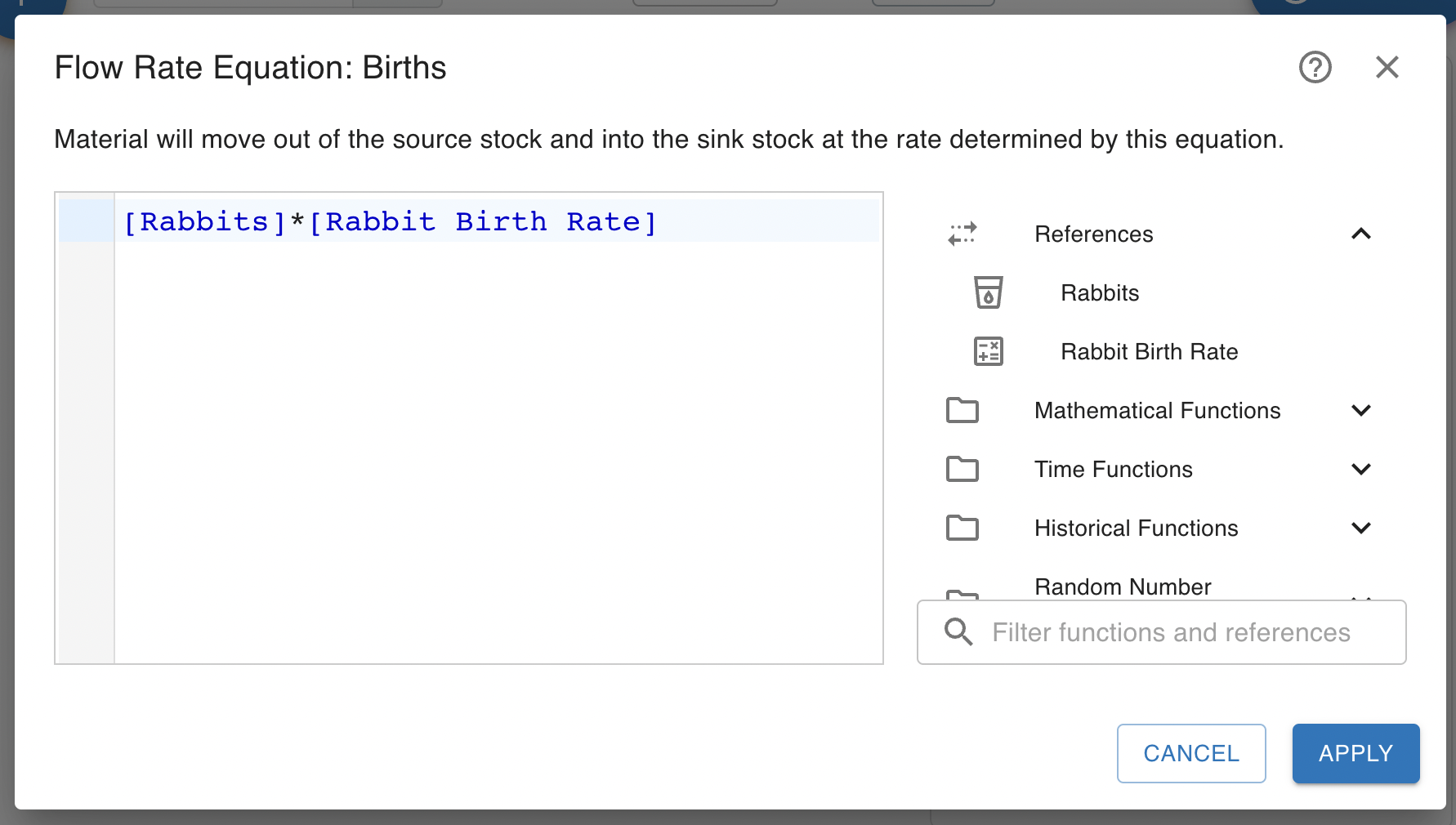# Equations and Formulas

The equation editor allows you to type in an equation. On the right of the editor are a list of primitives this primitive can reference and a list functions built into Insight Maker. You can click on one of these to insert it into the equation.These equations can be arbitrary mathematical or programming expressions. For instance, take the example of the following equation:

Sin(Years()*2) + [Rain Flow]^0.05

This example displays a number of features that the Insight Maker equation engine supports:

• ‘Years’ refers to the current simulation time as measured in years.
• ‘[Rain Flow]’ refers to the value of another primitive. Primitives can reference each other using this square-bracket notation.
• ‘Sin’ applies the trigonometric sine function.
• ‘+’, ‘*’, and ‘^’ are all standard mathematical operators representing addition, multiplication and exponentiation respectively.

There are a wide range of built-in functions you can use to develop equations.

You may also use units within equations. You can add units to number by surrounding the number and units with curly brackets "{" "}". For example:

{1 kilometer} + {100 meters}

Common units will automatically be converted in calculations, so the above will evaluate to:

{1.1 kilometers}

You can also combine units with "*", "/" or "^". For example, you could define 60 miles per hour like this example:

{60 miles/hours} * {10 minutes}